#### Risk Disclosure

Futures, foreign currency and options trading contains substantial risk and is not for every investor. An investor could potentially lose all or more than the initial investment. Risk capital is money that can be lost without jeopardizing ones financial security or lifestyle. Only risk capital should be used for trading and only those with sufficient risk capital should consider trading. Past performance is not necessarily indicative of future results. View full risk disclosure.

## Slope Solver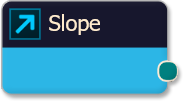The Slope solver offers multiple solutions for analyzing slope conditions.  e.g.  The Slope solver can identify if an indicator is sloping steeply, some what flat, or a moderate slope.

# BloodHound   v2

This section is specific to BloodHound v2.  Scroll down for BloodHound v1.

## Properties tab

This tab contains the essential settings for all nodes.

### Output Modifiers

This section provides quick access to commonly used output modifiers.
Please note:   The buttons only modify the individual instance of the selected node(s) on a Logic Board.   Thus, the buttons are only visible when a node is selected on the Logic Board.  The buttons are not available when a solver is selected in the Solvers panel, because the original solver's output can not be globally modified.
This behavior is consistent with adding a function node afterwards, so that the original solver's output remains unmodified elsewhere in the system.  It is similar to having an SMA(50) on several charts.  Changing the plot color on one chart does not modify the plot color on the other charts.

### Global Properties

These setting apply to all instances of the selected solver.  Changing a setting in one of the instances changes the setting in all instances.  If you only want to change one of the solvers, but not the others, you must make a copy of the solver.  The copy will be a different solver, and a number will be added at the end of the name to differentiate it from the original.

### Behavior

This option determines which outputs are calculated.  Effectively turning off either the Long or Short output.

#### Input

This section determines what kind of data the solver will evaluate.  Use the Type drop-down menu to select the data type.
The menu will change based on which data type is selected. Click on Type to see the various data types available and the corresponding documentation.

This menu selects the data type to be evaluated.  See below for detailed information on each data type.

##### Type:   Price

This option allows price data to be used in the solver.  The price data can be shaped/manipulated by the various settings before it is used by the solver.

Custom Prices

Body Top:  Regardless of the bar direction, this returns the higher price of the candlestick body.  Either the close or open price.  Whichever is on top.  e.g.  For an up bar, the Body Top equals the close price.
Body Median:    This returns the median price of the candlestick body.  The formula is (Close + Open) ÷ 2.
Body Bottom:    Regardless of the bar direction, this returns the lower price of the candlestick body.  Either the close or open price.  Whichever is the bottom.  e.g.  For an up bar, the Body Bottom equals the open price.

##### Type:   Volume

Volume will feed the current bar volume into the solver.
To evaluate the volume from a few bars back, use Indicator Value.  Then select the VOL indicator, and use the Displacement setting.

##### Type:   Swing Point Prices

Swing Point Prices uses the Swing Highs & Lows indicator plots for input price data.  This is useful when you need to access the lowest low or highest high prices from the latest swing point.
You can add the bundled indicator Swing Highs & Lows to the chart to help you visualize the price data the solver is using.

## Options tab

This tab contains extra secondary settings common to all nodes.

### Weight

This section is used to modify the node's final output value.  It is only useful for designing fuzzy logic systems, or a fuzzy logic section of a system.
Please note:   The Weight controls only modify the individual instance of the selected node(s) on a Logic Board.   Thus, the Weight controls are only visible when a node is selected on the Logic Board.  The controls are not available when a solver is selected in the Solvers panel, because the original solver's output can not be globally modified.
This behavior is different than BloodHound 1.0.  In BH 1.0, the Weight controls modified the nodes output globally (every instance).  This change in BH 2.0 adds more system design granularity.

Multiply Output:   This takes the internal values (the Long and Short values determined via the Properties tab » Output Rules section) and multiplies them by this value.  Note: the final output will not exceed a value of 1, as described in the Slider control below.

Slider control:  The slider constrains the final output to a value of 0 to 1.  The left side of the slider sets a minimum value that is output regardless if the solver condition is true or not.  The right side sets a maximum value that is output.  The output is capped.
e.g.  Three indicator conditions are being checked, and thus three solvers are created.  Only two out of the three indicator conditions are needed.  An Additive logic node is used to add the solver's outputs together.  Just two out of the three solvers need to add up to a value of 1.  Therefore, the right slider (max output value) for all three solvers is set to 0.5.  When two indicator/solver conditions are found, thus the outputs = 0.5, then the calculation, in the Additive node, is 0.5 + 0.5 + 0 = 1.  A value of 1 means the two out of three condition is true.

Hedge Operation: This applies a mathematical formula to the internal value.
None:  No modification is applied.
Very (square output):  A squaring formula is applied.  Output  =  value .
Somewhat (square root output):  A square root formula is applied.  Output  =  √value .

### Description

This text area provides a place to write a full description of what the node is doing, used for, or what ever you want.
Note:   The Description is global to all instances of the node.  It is not applied individually to each instance as the Weight controls are.

## Help tab

This tab displays the documentation page (from this web site) of the selected node.
Please note:  NinjaTrader v8.0.26.0 or newer is required for the built in web viewer to work, and thus the documentation to be displayed.

### Video Tutorial

This video is from our weekly Workshop in July 2015.
For more benefit please watch in full screen mode, as this video is recorded in HD.

This video shows BloodHound v1, however the concepts and principals are still the same.

### Examples

The screenshots below show BloodHound v1, however the concepts and principals are still the same.

#### Example 1A: The Basics

This demonstrates the basic function of Indicator Slope solver with the use of the Stochastic D.  This Solver will be used to detect which direction the Stochastic is pointing by analyzing if the slope is positive or negative.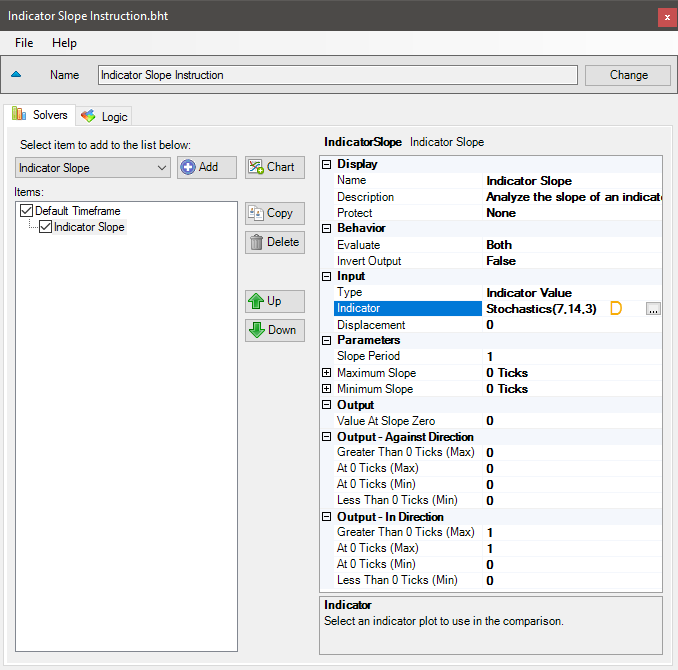• Add the Indicator Slope solver
• Set Indicator to Stochastic D

The chart below shows a Long signal when the Stochastic D is pointing up, and a Short signal when it is pointing down.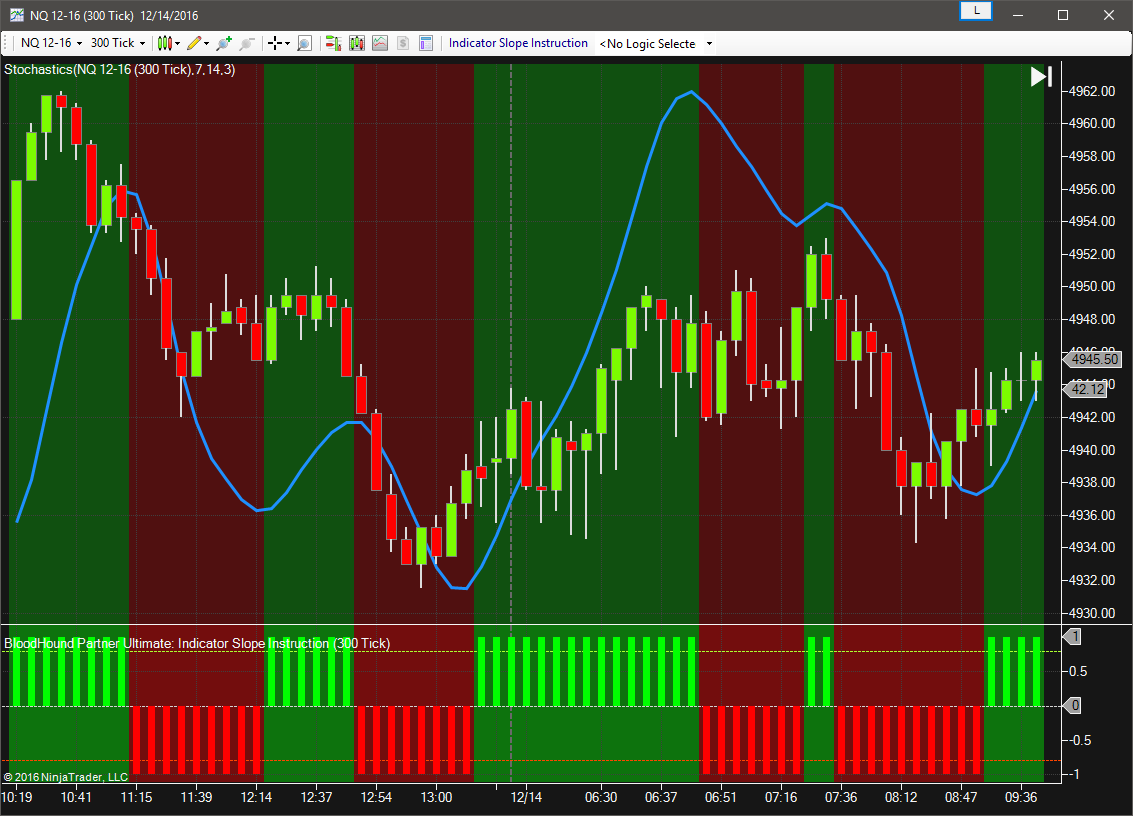#### Example 1B: The Basics with Filtering

This demonstrates the basic function of Indicator Slope solver as an indicator angle filter.  This Solver will be used to detect strong angles in the SMA 14.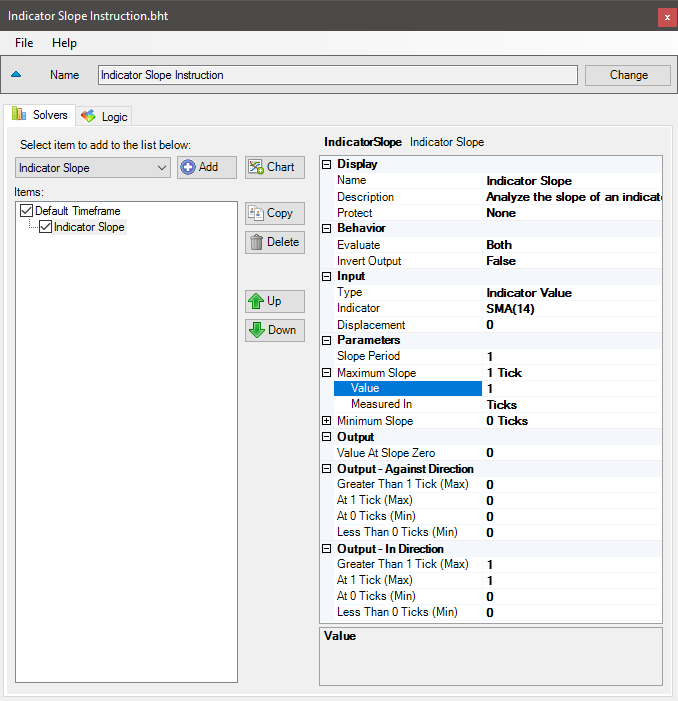• Add the Indicator Slope solver
• Set Indicator to SMA
• Set Maximum Slope to 1 Ticks

As the chart below shows, when the angle of the SMA 14 is strong in either direction a signal is given.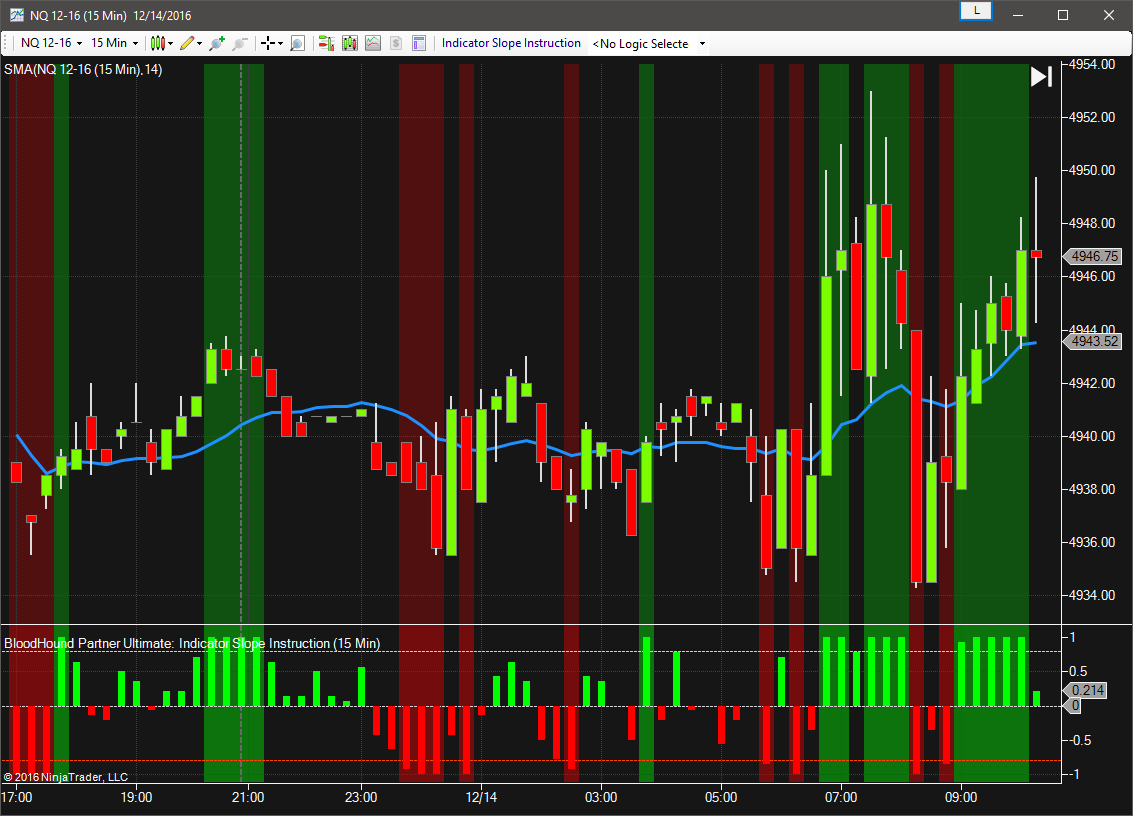#### Example 2A: Finding Flatness (or Consolidation)

This demonstrates how to find price consolidation with the Indicator Slope solver. This Solver will be used to detect flat areas in the SMA 14 and generate a bi-directional signal that can be used in an exit system.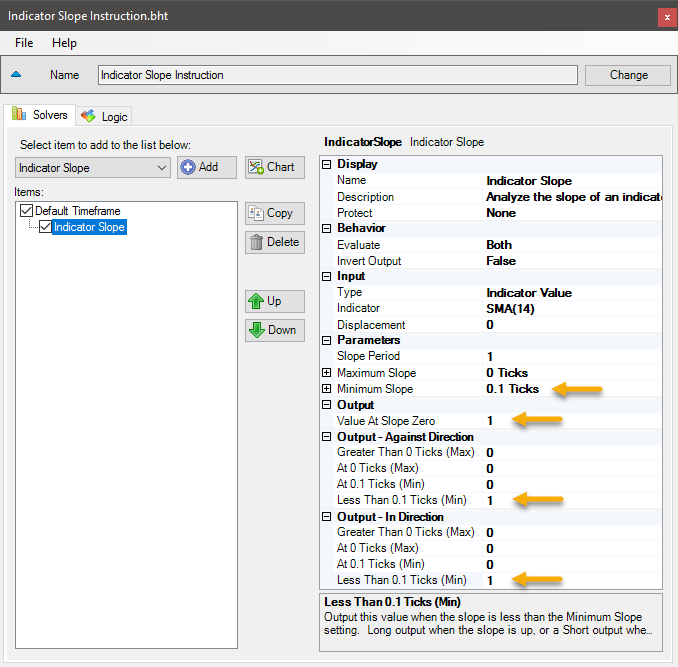• Add the Indicator Slope solver
• Set Indicator to SMA
• Set Minimum Slope to 0.1 Ticks
• Set Output Value At Slope Zero to 1
• Set Output-Against Direction Less Than Min to 1
• Set Output-In Direction Greater Than Max to 0
• Set Output-In Direction At Max to 0
• Set Output-In Direction Less Than Min to 1

The chart below shows, when the SMA 14 is flat(ish) a bi-directional signal is given. A bi-directional output was setup, because a flat SMA is neither Long or Short.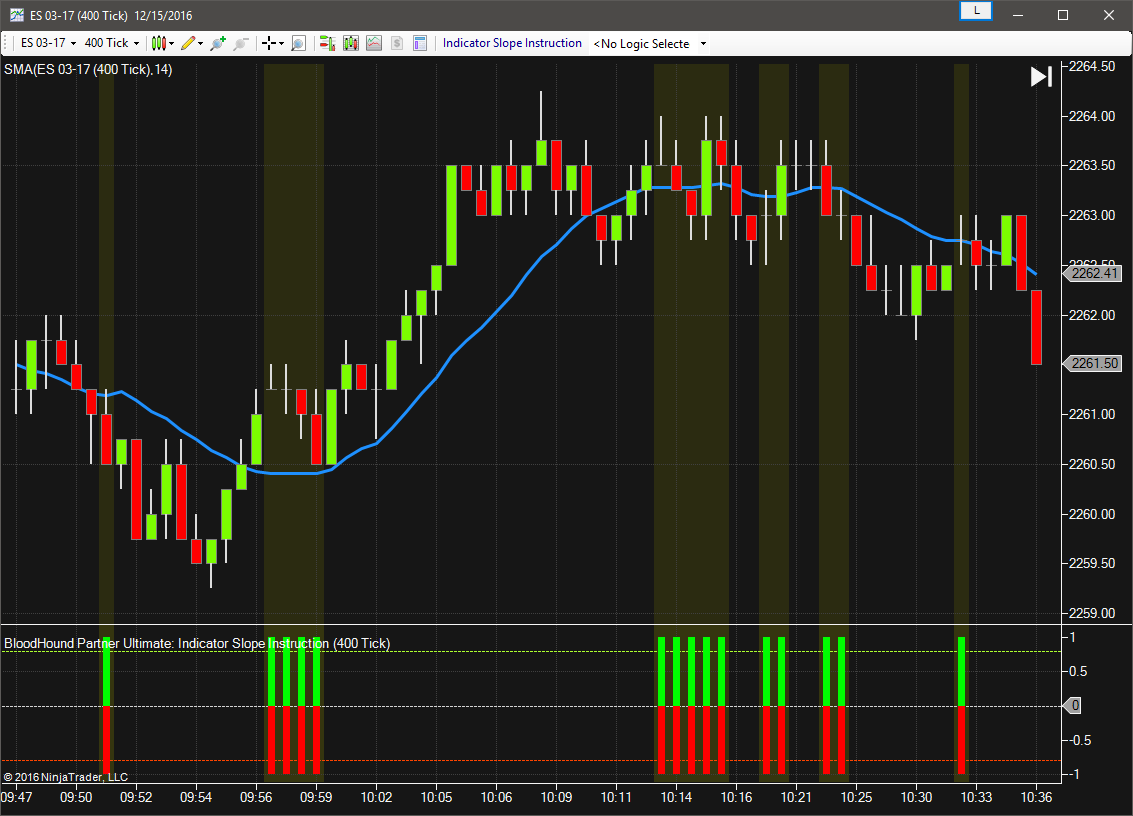#### Example 2B: Finding Consolidation with ADXVMA

This demonstrates a specialized way to find price consolidation with the Indicator Slope solver. This time we’ll use the SiADXVMA indicator (a similar indicator is avilable from Lizard Trader) to determine consolidation and generate a bi-directional signal that can be used in an exit system.
<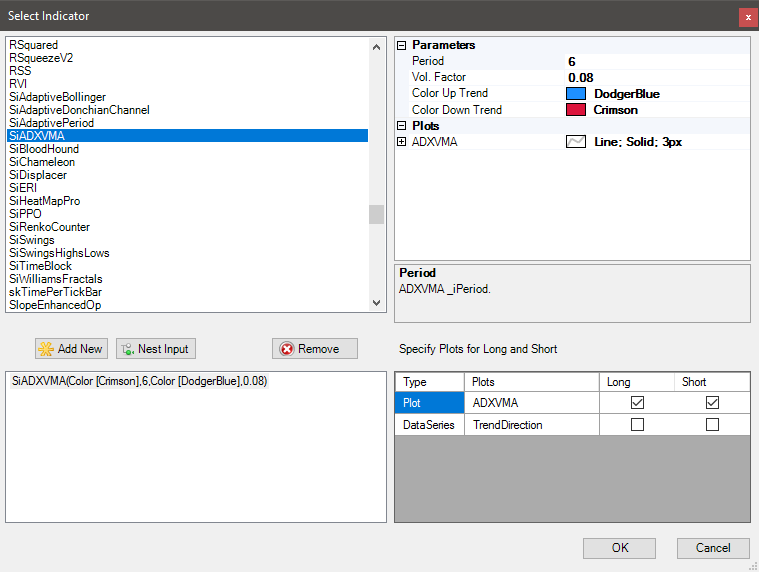• Add the Indicator Slope solver
• Set Indicator to SiADXVMA
• Set the Period to 6
• Select DataSeries ADXVMA: Long and Short checkbox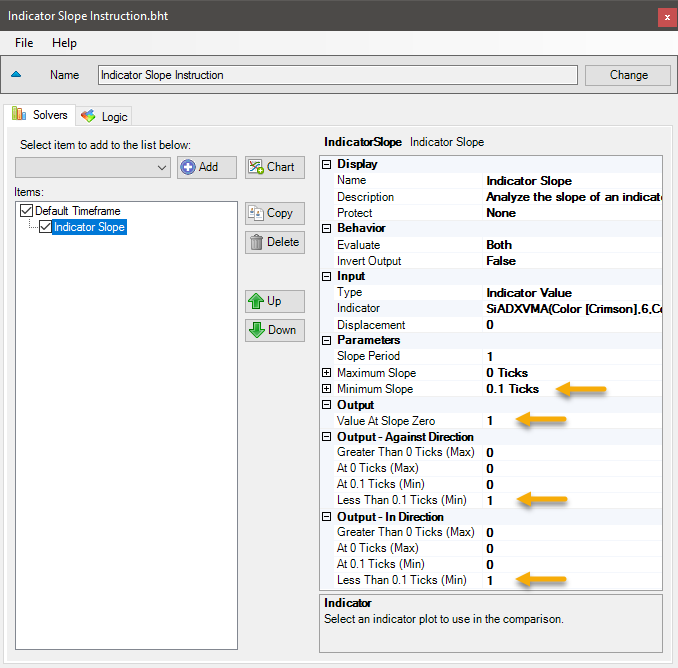• Set Minimum Slope to 0.1 Ticks
• Set Output Value At Slope Zero to 1
• Set Output-Against Direction Less Than Min to 1
• Set Output-In Direction Greater Than Max to 0
• Set Output-In Direction At Max to 0
• Set Output-In Direction Less Than Min to 1

The chart below shows, when the ADXVMA is flat a bi-directional signal is given. A bi-directional output was setup, because a flat ADXVMA is neither Long or Short.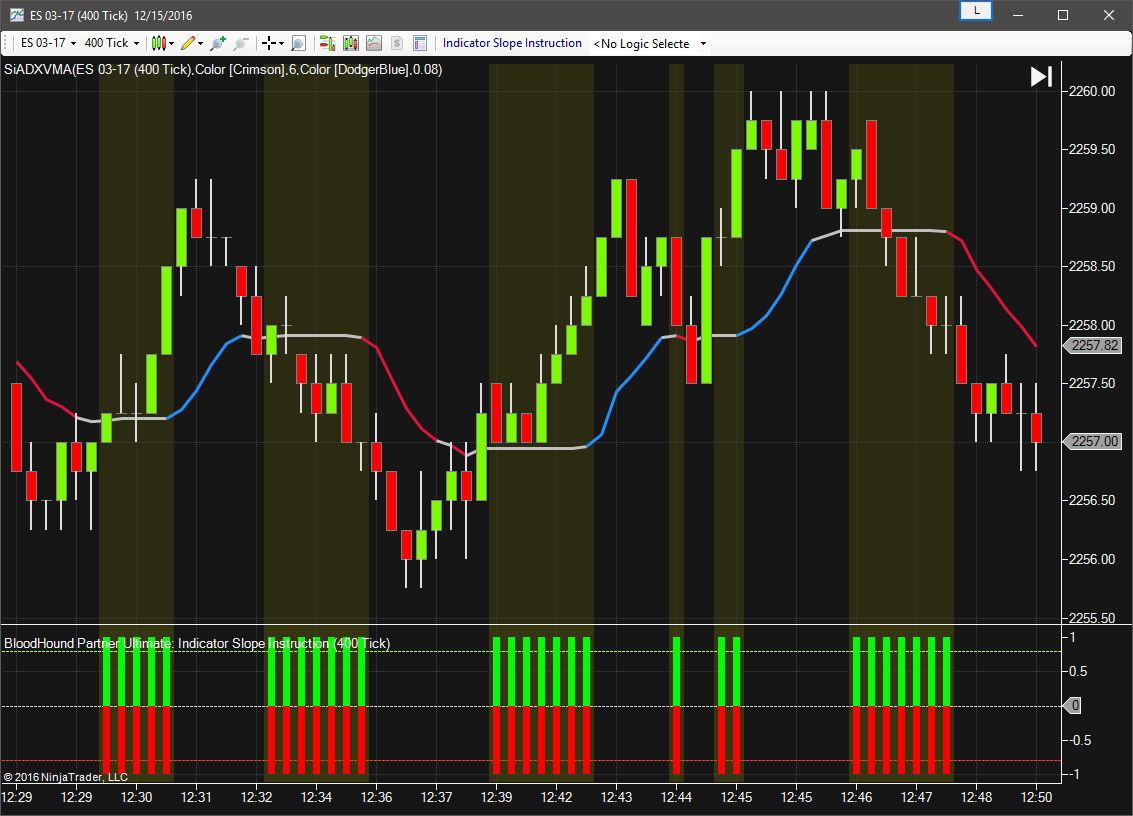#### Risk Disclosure

Futures, foreign currency and options trading contains substantial risk and is not for every investor. An investor could potentially lose all or more than the initial investment. Risk capital is money that can be lost without jeopardizing ones financial security or lifestyle. Only risk capital should be used for trading and only those with sufficient risk capital should consider trading. Past performance is not necessarily indicative of future results. View full risk disclosure.

# BloodHound   v1

This section is specific to BloodHound v1.  Scroll up for BloodHound v2.

# Indicator Slope SolverThe Slope solver offers multiple solutions for analyzing slope conditions, and user definable signals (output).

### Video Tutorial

This video is from our weekly Workshop in July 2015.
For more benefit please watch in full screen mode, as this video is recorded in HD.

### Examples

#### Example 1A: The Basics

This demonstrates the basic function of Indicator Slope solver with the use of the Stochastic D.  This Solver will be used to detect which direction the Stochastic is pointing by analyzing if the slope is positive or negative.• Add the Indicator Slope solver
• Set Indicator to Stochastic D

The chart below shows a Long signal when the Stochastic D is pointing up, and a Short signal when it is pointing down.#### Example 1B: The Basics with Filtering

This demonstrates the basic function of Indicator Slope solver as an indicator angle filter.  This Solver will be used to detect strong angles in the SMA 14.• Add the Indicator Slope solver
• Set Indicator to SMA
• Set Maximum Slope to 1 Ticks

As the chart below shows, when the angle of the SMA 14 is strong in either direction a signal is given.#### Example 2A: Finding Flatness (or Consolidation)

This demonstrates how to find price consolidation with the Indicator Slope solver. This Solver will be used to detect flat areas in the SMA 14 and generate a bi-directional signal that can be used in an exit system.• Add the Indicator Slope solver
• Set Indicator to SMA
• Set Minimum Slope to 0.1 Ticks
• Set Output Value At Slope Zero to 1
• Set Output-Against Direction Less Than Min to 1
• Set Output-In Direction Greater Than Max to 0
• Set Output-In Direction At Max to 0
• Set Output-In Direction Less Than Min to 1

The chart below shows, when the SMA 14 is flat(ish) a bi-directional signal is given. A bi-directional output was setup, because a flat SMA is neither Long or Short.#### Example 2B: Finding Consolidation with ADXVMA

This demonstrates a specialized way to find price consolidation with the Indicator Slope solver. This time we’ll use the SiADXVMA indicator (a similar indicator is avilable from Lizard Trader) to determine consolidation and generate a bi-directional signal that can be used in an exit system.
<• Add the Indicator Slope solver
• Set Indicator to SiADXVMA
• Set the Period to 6
• Select DataSeries ADXVMA: Long and Short checkbox• Set Minimum Slope to 0.1 Ticks
• Set Output Value At Slope Zero to 1
• Set Output-Against Direction Less Than Min to 1
• Set Output-In Direction Greater Than Max to 0
• Set Output-In Direction At Max to 0
• Set Output-In Direction Less Than Min to 1

The chart below shows, when the ADXVMA is flat a bi-directional signal is given. A bi-directional output was setup, because a flat ADXVMA is neither Long or Short.#### Risk Disclosure

Futures, foreign currency and options trading contains substantial risk and is not for every investor. An investor could potentially lose all or more than the initial investment. Risk capital is money that can be lost without jeopardizing ones financial security or lifestyle. Only risk capital should be used for trading and only those with sufficient risk capital should consider trading. Past performance is not necessarily indicative of future results. View full risk disclosure.

## Slope SolverThe Slope solver offers multiple solutions for analyzing slope conditions, and user definable signals (output).

### Video Tutorial

This video is from our weekly Workshop in July 2015.
For more benefit please watch in full screen mode, as this video is recorded in HD.

### Examples

#### Example 1A: The Basics

This demonstrates the basic function of Indicator Slope solver with the use of the Stochastic D.  This Solver will be used to detect which direction the Stochastic is pointing by analyzing if the slope is positive or negative.• Add the Indicator Slope solver
• Set Indicator to Stochastic D

The chart below shows a Long signal when the Stochastic D is pointing up, and a Short signal when it is pointing down.#### Example 1B: The Basics with Filtering

This demonstrates the basic function of Indicator Slope solver as an indicator angle filter.  This Solver will be used to detect strong angles in the SMA 14.• Add the Indicator Slope solver
• Set Indicator to SMA
• Set Maximum Slope to 1 Ticks

As the chart below shows, when the angle of the SMA 14 is strong in either direction a signal is given.#### Example 2A: Finding Flatness (or Consolidation)

This demonstrates how to find price consolidation with the Indicator Slope solver. This Solver will be used to detect flat areas in the SMA 14 and generate a bi-directional signal that can be used in an exit system.• Add the Indicator Slope solver
• Set Indicator to SMA
• Set Minimum Slope to 0.1 Ticks
• Set Output Value At Slope Zero to 1
• Set Output-Against Direction Less Than Min to 1
• Set Output-In Direction Greater Than Max to 0
• Set Output-In Direction At Max to 0
• Set Output-In Direction Less Than Min to 1

The chart below shows, when the SMA 14 is flat(ish) a bi-directional signal is given. A bi-directional output was setup, because a flat SMA is neither Long or Short.#### Example 2B: Finding Consolidation with ADXVMA

This demonstrates a specialized way to find price consolidation with the Indicator Slope solver. This time we’ll use the SiADXVMA indicator (a similar indicator is avilable from Lizard Trader) to determine consolidation and generate a bi-directional signal that can be used in an exit system.
<• Add the Indicator Slope solver
• Set Indicator to SiADXVMA
• Set the Period to 6
• Select DataSeries ADXVMA: Long and Short checkbox• Set Minimum Slope to 0.1 Ticks
• Set Output Value At Slope Zero to 1
• Set Output-Against Direction Less Than Min to 1
• Set Output-In Direction Greater Than Max to 0
• Set Output-In Direction At Max to 0
• Set Output-In Direction Less Than Min to 1

The chart below shows, when the ADXVMA is flat a bi-directional signal is given. A bi-directional output was setup, because a flat ADXVMA is neither Long or Short.